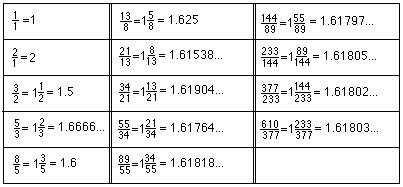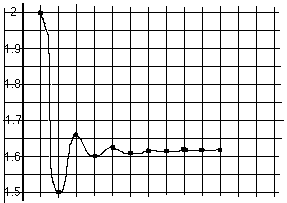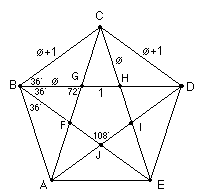## Answers for Chapter 7 Sample Problems

2. A program to get the Fibonacci numbers

• 10 x=1
• 20 PRINT x
• 30 y=1
• 40 PRINT y
• 50 s=x+y
• 60 PRINT s
• 70 x=y
• 80 y=s
• 90 GOTO 50

3. The Fibonacci ratios:The graph of the Fibonacci ratios:The number this infinite sequence approaches is The Golden Mean or The Divine Proportion. It is an irrational number and the positive solution of the quadratic equation x2-x-1=0, which is (1+Sqrt(5))/2, about 1.618034. The Greek letter 'phi' (see below for the symbol) is sometimes used to represent this number.
2. The regular pentagonBack to Ch. 7 problems
To order Don's materials
To choose sample problems from other chapters
Mathman home